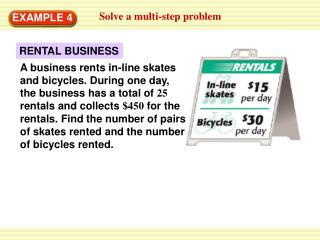DownloadDownload PresentationEXAMPLE 4

# EXAMPLE 4

Télécharger la présentation## EXAMPLE 4

- - - - - - - - - - - - - - - - - - - - - - - - - - - E N D - - - - - - - - - - - - - - - - - - - - - - - - - - -
##### Presentation Transcript

1. Solve a multi-step problem EXAMPLE 4 RENTAL BUSINESS A business rents in-line skates and bicycles. During one day, the business has a total of 25 rentals and collects \$450 for the rentals. Find the number of pairs of skates rented and the number of bicycles rented.

2. Solve a multi-step problem EXAMPLE 4 SOLUTION STEP 1 Write a linear system. Let xbe the number of pairs of skates rented, and let ybe the number of bicycles rented. x + y = 25 Equation for number of rentals 15x + 30y = 450 Equation for money collected from rentals STEP2 Graph both equations.

3. ANSWER The business rented 20 pairs of skates and 5 bicycles. ? ? 15(20) + 30(5) 450 20+5 25 = = 450 = 450 25 = 25 Solve a multi-step problem EXAMPLE 4 STEP 3 Estimate the point of intersection. The two lines appear to intersect at(20, 5). STEP 4 Check whether (20, 5) is a solution.

4. ANSWER In Example 4, suppose the business has a total of 20 rentals and collects \$420. Find the number of bicycles rented. 6. 8 bicycles Solve a multi-step problem EXAMPLE 4 for Example 4 GUIDED PRACTICE